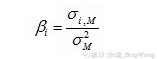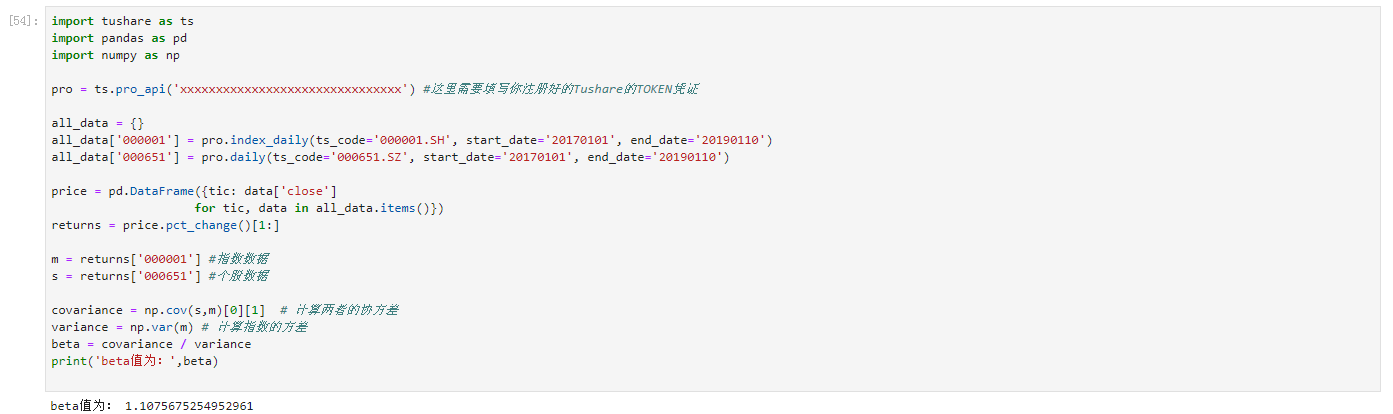# 贝塔值（beta）的线性回归方法实践#

## 线性回归(Linear Regression)#1. 我们首先调用Tushare的API 获取股票数据；

2. 再通过python计算出其股票价格每日的百分比变化；

3. 利用python中的numpy库计算协方差和方差值；

4. 最后计算贝塔值（beta）。

## 调用Tushare的API 获取股票数据#

import tushare as ts
import pandas as pd

pro = ts.pro_api('xxxxxxxxxxxxxxxxxxxxxxxxxxxxxxx') #这里需要填写你注册好的Tushare的TOKEN凭证


#先建立一个字典，用来存储股票、指数对应的价格
all_data = {}

#获取大盘5年的日线数据
all_data['000001'] = pro.index_daily(ts_code='000001.SH', start_date='20170101', end_date='20190110')
#获取个股5年的日线数据
all_data['000651'] = pro.daily(ts_code='000651.SZ', start_date='20170101', end_date='20190110')


## 计算日线数据百分比变化#

#用for循环提取股票、大盘的收盘价格并转换为dataframe的形式
price = pd.DataFrame({tic: data['close']
for tic, data in all_data.items()})
#计算股票价格每日变化
returns = price.pct_change()[1:]



## 计算协方差和方差值#

import numpy as np

m = returns['000001.SH'] #指数数据
s = returns['000651.SZ'] #个股数据

covariance = np.cov(s,m)  # 计算两者的协方差
variance = np.var(m) # 计算指数的方差


## 计算贝塔值（beta）#

beta = covariance / variance
print(beta)


## 完整代码#

import tushare as ts
import pandas as pd
import numpy as np

pro = ts.pro_api('xxxxxxxxxxxxxxxxxxxxxxxxxxxxxxx') #这里需要填写你注册好的Tushare的TOKEN凭证

all_data = {}
all_data['000001'] = pro.index_daily(ts_code='000001.SH', start_date='20140101', end_date='20190110')
all_data['000651'] = pro.daily(ts_code='000651.SZ', start_date='20140101', end_date='20190110')

price = pd.DataFrame({tic: data['close']
for tic, data in all_data.items()})
returns = price.pct_change()[1:]

m = returns['000001'] #指数数据
s = returns['000651'] #个股数据

covariance = np.cov(s,m)  # 计算两者的协方差
variance = np.var(m) # 计算指数的方差
beta = covariance / variance
print('beta值为：',beta)



## 结果#